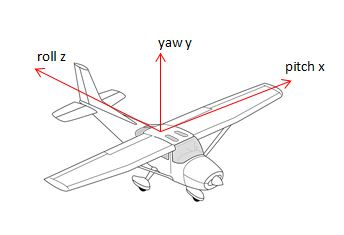# Rotation Conventions

## Rotation Conventions

There are several methods of achieving rotations within Babylon.js all of which use a particular convention.

## Euler Angles

In 3D space Euler angles can produce any possible orientation by providing three angles to rotate about each of three axes in a given order.

For three axes X, Y and Z there are 12 different permutations for the order of the angles. Since X, Y and Z can be in World Space or in Local Space this means there is a potential of 24 different possibilities. Most, if not all,of these are in use in different systems around the world. So you need to be very careful that you know very clearly the convention that the system you are working in uses.

Mesh.rotation(alpha, beta, gamma) uses the three Euler angles alpha, beta and gamma which are rotations about the X, Y and Z axes respectively. The convention that Babylon.js uses is based on the yaw, pitch and roll convention and so is carried out around X, Y and Z in local space in the order Y, X, Z.

References to Euler angles within the Babylon.js community can usually be taken to mean the angles to use with the rotation method.

### YXZ Local Axes Yaw, Pitch, RollA pitch is about X, yaw about Y and roll about Z applied in the order yaw, pitch roll using local axes.

Applying independent rotations to a newly created mesh (ie one that has zero rotations) in the order YXZ using local axes

mesh.rotate(BABYLON.Axis.Y, yaw, BABYLON.Space.LOCAL);mesh.rotate(BABYLON.Axis.X, pitch, BABYLON.Space.LOCAL);mesh.rotate(BABYLON.Axis.Z, roll, BABYLON.Space.LOCAL);

produces the same orientation as

mesh.rotation = new BABYLON.Vector3(pitch, yaw, roll);

which will produce this orientation whatever the orientation of the mesh prior to its application. The playground below demonstrates this by randomly generating angles and then applying these two methods to two different boxes which remain in alignment.

YXZ Yaw, Pitch, Roll

### ZXY World Axes

The YXZ convention with local axes has produced a particular orientation and it turns out that taking the same angles (alpha = pitch, beta = yaw and gamma = roll) and applying them in the order ZXY in world space will produce exactly the same orientation.

Applying independent rotations to a newly created mesh (ie one that has zero rotations) in the order ZXY

mesh.rotate(BABYLON.Axis.Z, gamma, BABYLON.Space.WORLD);mesh.rotate(BABYLON.Axis.X, alpha, BABYLON.Space.WORLD);mesh.rotate(BABYLON.Axis.Y, beta, BABYLON.Space.WORLD);

produces the same orientation as

mesh.rotation = new BABYLON.Vector3(alpha, beta, gamma);

which will produce this orientation whatever the orientation of the mesh prior to its application. The playground below demonstrates this by randomly generating angles and then applying these two methods to two different boxes which remain in alignment.

ZXY Example

## Euler Angles to Quaternions

### YXZ, Local Space, Yaw, Pitch, RollAs a reminder this convention is directly used in the rotation method in Babylon.js in the form

mesh.rotation = new BABYLON.Vector3(pitch, yaw, roll);

In this convention to take the three angles, yaw, pitch and roll and rotate rotate yaw about Y, then pitch about X and roll about Z using the local axes.

mesh.rotate(BABYLON.Axis.Y, yaw, BABYLON.Space.LOCAL);mesh.rotate(BABYLON.Axis.X, pitch, BABYLON.Space.LOCAL);mesh.rotate(BABYLON.Axis.Z, roll, BABYLON.Space.LOCAL);

which with quaternions is the same as using RotationYawPitchRoll

const yprQuaternion = BABYLON.Quaternion.RotationYawPitchRoll(yaw, pitch, roll);

Applying the above rotate sequence to a newly created mesh (ie one that has zero rotations) in the order YXZ in local space and applying RotationAlphaBetaGamma to a mesh, with any orientation, using the same angles will produce the same orientation. The playground below demonstrates this by randomly generating angles and then applying these two methods to two different boxes which remain in alignment.

Yaw Pitch Roll to Quaternion

### ZXZ, World Space, A Standard Convention

A standard Euler angle convention is to take three given angles alpha, beta and gamma and rotate alpha about Z, then beta about X, then gamma about Z using the world axes. In Babylon.js this can be achieved by using rotate

mesh.rotate(BABYLON.Axis.Z, alpha, BABYLON.Space.WORLD);mesh.rotate(BABYLON.Axis.X, beta, BABYLON.Space.WORLD);mesh.rotate(BABYLON.Axis.Z, gamma, BABYLON.Space.WORLD);

which with quaternions is the same as using RotationAlphaBetaGamma

var abcQuaternion = BABYLON.Quaternion.RotationAlphaBetaGamma(alpha, beta, gamma);

Applying the above rotate sequence to a newly created mesh (ie one that has zero rotations) in the order ZXZ in world space and applying RotationAlphaBetaGamma to a mesh, with any orientation, using the same angles will produce the same orientation. The playground below demonstrates this by randomly generating angles and then applying these two methods to two different boxes which remain in alignment.

ZXZ To Quaternion

So far we have considered one mesh operating in its local space and the world space of Babylon.js. What if we want one mesh to operate in the frame of reference of another mesh. Before we consider parenting, in the next section, we will look at ways this can be done using the data that Babylon.js directly stores on the transformation of a mesh and its coordinates.